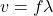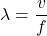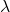## A guitar string vibrates at a frequency of 330Hz with wavelength 1.40m. The frequency and wavelength of this sound wave in air (20 C) when i

Question

A guitar string vibrates at a frequency of 330Hz with wavelength 1.40m. The frequency and wavelength of this sound wave in air (20 C) when it reaches our ears is: Lower frequency, same wavelength Same frequency, same wavelength Higher frequency, same wavelength Same frequency, shorter wavelength

in progress 0
4 months 2021-07-14T04:14:54+00:00 1 Answers 17 views 0

Same frequency, shorter wavelength

Explanation:

The speed of a wave is given bywhere,

f = Frequency= Wavelength

It can be seen that the wavelength is directly proportional to the velocity.

Here the frequency of the sound does not change.

But the velocity of the sound in air is slower.

Hence, the frequency remains same and the wavelength shortens.